# KSEEB SSLC Class 10 Maths Solutions Chapter 11 Introduction to Trigonometry Ex 11.1

In this chapter, we provide KSEEB SSLC Class 10 Maths Solutions Solutions Chapter 11 Introduction to Trigonometry Ex 11.1 for English medium students, Which will very helpful for every student in their exams. Students can download the latest KSEEB SSLC Class 10 Maths Solutions Solutions Chapter 11 Introduction to Trigonometry Ex 11.1 pdf, free KSEEB SSLC Class 10 Maths Solutions Solutions Chapter 11 Introduction to Trigonometry Ex 11.1 pdf download. Now you will get step by step solution to each question.

### Karnataka State Syllabus Class 10 Maths SolutionsChapter 11 Introduction to Trigonometry Ex 11.1

Trigonometry Exercise 11.1 Solutions Question 1.
In ∆ABC, right-angled at B, AB = 24 cm., BC = 7 cm. Determine:
i) sin A, cos A
ii) sin C, cos C
Solution: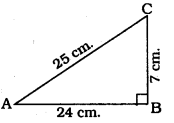In ⊥∆ABC, ∠B = 90°
As per Pythagoras theorem
AC2 = AB2 + BC2
= (24)2 + (7) 2
= 576 + 49
AC2 = 625
∴ AC = 25 cm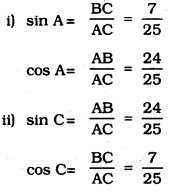Introduction To Trigonometry Class 10 Exercise 11.1 Question 2.
In the given figure, find tan P – cot R.
Solution: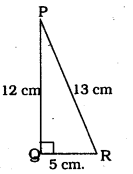In ⊥∆PQR, ∠Q = 90°
∴ PQ2 + QR2 = PR2
(12)2 + QR2 = (13)2
144 + QR2 = 169
QR2 = 169 – 144
QR2 = 25
∴ QR = 5 cm.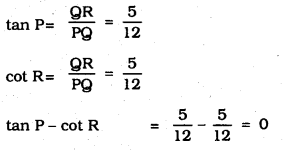Introduction To Trigonometry Exercise 11.1 Question 3.
If sin A = 34 calculate cos A and tan A.
Solution: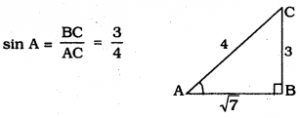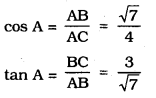Exercise 11.1 Class 10 Trigonometry Question 4.
Given 15 cot A = 8, find sin A and sec A.
Solution: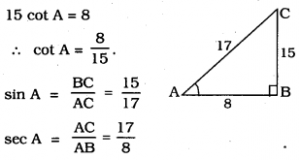KSEEB Solutions For Class 10 Maths Trigonometry Question 5.
Given sec θ = 1312, calculate all other trigonometric ratios
Solution;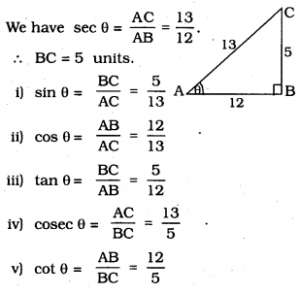10th Maths Introduction To Trigonometry Exercise 11.1 Question 6.
If ∠A and ∠B are acute angles such that cos A = cos B, then show that ∠A = ∠B.
Solution:
In ⊥∆ABC, ∠A and ∠B are acute angles. CD ⊥ AB is drawn.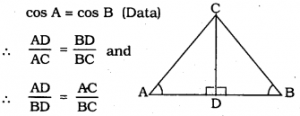CD is common.
S.S.S. Postulate :
∴ ∠A = ∠B
∵ “Angles of similar triangles are equiangular.”

10th Class Maths Trigonometry Exercise 11.1 Question 7.
If cot θ = 78, evaluate :
(i) (1+sinθ)(1−sinθ)(1+cosθ)(1−cosθ)
(ii) cot2θ
Solution: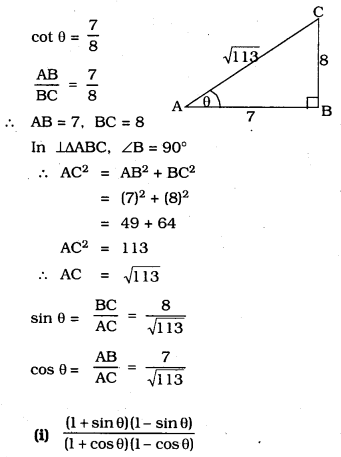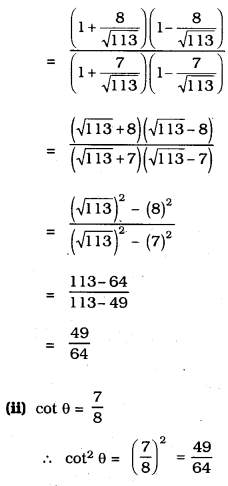Trigonometry Class 10 Exercise 11.1 Question 8.
If 3 cot A = 4, check whether 1−tan2A1+tan2A = cos2 A – sin2 A or not
Solution: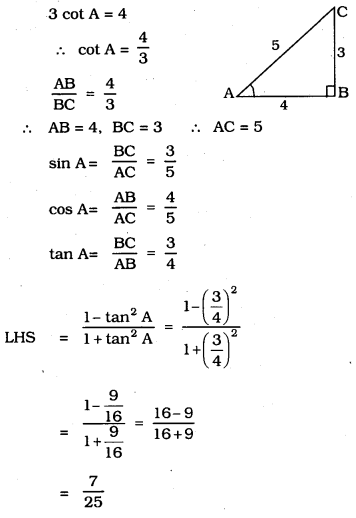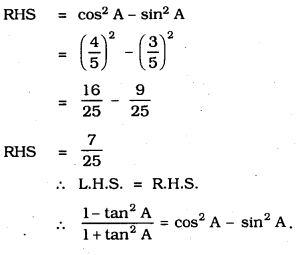Introduction To Trigonometry Class 10 KSEEB Question 9.
In ∆ABC, right-angled at B, if tan A =13√ find the value of:
i) sin A cos C + cos A sin C
ii) cos A cos C – sin A sin C
Solution: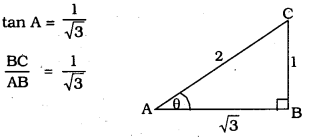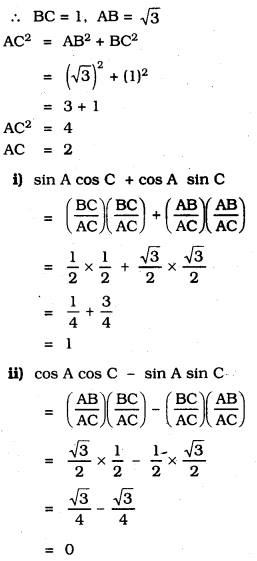Trigonometry Ex 11.1 Question 10.
In ∆PQR, right-angled at Q, PR + QR = 25 cm. and PQ = 5 cm. Determine the values of sin P, cos P and tan P
Solution:
PQ = 5 cm
PR + QR = 25 cm
∴ PR = 25 – QR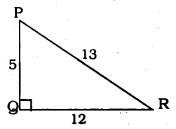PR2 = PQ2 + QR2
QR2 = PR2 – PQ2
= (25 – QR)2 – (5)2
QR2 = 625 – 50QR + QR2 – 25
50QR = 600
∴ QR = 12 cm.
∴ PR = 25 – QR = 25 – 12 = 13 cm.
∴ QR = 12 cm
∴ PR = 25 – QR = 25 – 12 = 13 cm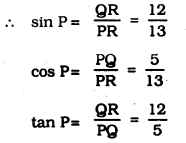10th Class Trigonometry Exercise 11.1 Question 11.
(i) The value of tan A is always less than 1.
False. 60° = 3–√ > 1

(ii) If sec A = 125 for some value of angle A.
True. because sec A > 1.

(iii) cos A is the abbreviation used for the cosecant of angle A.
False. Because cos A is simplified as cos.

(iv) cot A is the product of cot and A.
False. cot is ∠A or meaningless. Here,
cot A =  Adjacent side  Opposite side

(v) sin θ = 43 for some angle θ
False. because sin θ ≯ 1

All Chapter KSEEB Solutions For Class 10 Maths

—————————————————————————–

All Subject KSEEB Solutions For Class 10

*************************************************

I think you got complete solutions for this chapter. If You have any queries regarding this chapter, please comment on the below section our subject teacher will answer you. We tried our best to give complete solutions so you got good marks in your exam.

If these solutions have helped you, you can also share kseebsolutionsfor.com to your friends.

Best of Luck!!